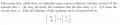# Eigen Vectors of 2 * 2 Matrix

#### zulfi100

Joined Jun 7, 2012
656
Hi,

I got following example from a web site:

Row1 = 2 2

Row2 = 5 -1

After finding the determinant, they got : lambda^2 -lambda -12 =0

&

lambda1 = -3 and lambda2 = 4

then tried to find eigen vectors : x1= -2/5 x2. After that they got one eigen vector

u1 = 2 -5

I cant understand how x1 equation is used for Eigen vector. If suppose x1 = -2x2 thenu1 = -2 1.
Is this correct? Some body please guide me

Zulfi.

#### drc_567

Joined Dec 29, 2008
1,156
https://en.m.wikipedia.org/wiki/Eigenvalues_and_eigenvectors

There is a useful illustration under the Matrix Example section ... see the active graph.

The eigenvalues act as multipliers for a given set of eigenvectors, and the square matrix is an operator or transformation on the entire group of vectors.